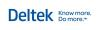You are here: Ajera Help (A-Z) > Inquiries > About creating formulas

When you create a new inquiry column, you can either add it from the predefined list of columns, or create your own column by defining a formula for it.

You can often write the formula you need by entering simple mathematical equations. However, to enter more complicated formulas, you must either understand how to use Microsoft SQL commands or request the services of an Ajera consultant (to learn more about Microsoft SQL, search the Microsoft website). If needed, you can also click the Properties button on the Inquiry toolbar and set conditions on the formula.

When you create a formula, you can use other existing formulas in the inquiry. These appear in the Available Columns area of the Formula Editor. The Available Columns area also contains the Current User Key, which allows you to display inquiry data pertaining only to the user.

You can create the following formulas in Inquiry:

• Basic formulas: You can create many inquiry formulas using basic mathematical operators. For example, here are formulas for two new columns on the Project inquiry:
[Billed] - [Cost]
([Billed] + [WIP]) - [Cost]
• Advanced formulas: When you write an advanced formula, you create a new column by defining relationships between columns that already exist in the inquiry. To create advanced formulas, you do not need to know the structure of the Ajera database. To write advanced formulas, you use the Microsoft SQL functions. Some commonly used functions in advanced formulas include:
• LEFT
• RIGHT
• SUBSTRING
• DATEDIFF
• MONTH
• UPPERCASE
• Here is an example of a formula for the Transaction - All inquiry.
 CASE [Activity Type] WHEN 'Labor' THEN [Employee] WHEN 'Expense' THEN [Activity] WHEN 'Consultant' THEN [Vendor] WHEN 'None' THEN 'Invoice Adjustment' END
• This formula uses the SQL function, CASE, to populate the new column as follows:

If the activity type is

Then the formula returns

Labor

the employee

Expense

the activity

Consultant

the vendor

None

• Advanced custom formulas: You may choose to write an advanced custom formula when you need to create an entirely new column that is not based on a relationship between existing columns. To write advanced custom formulas, you must know the structure of the Ajera database and use Microsoft SQL functions. For up-to-date database schema information, refer to the AxSchema spreadsheet in your Ajera\Program folder. Some commonly used functions in advanced custom formulas include:
• SELECT
• FROM
• WHERE
• A good use of an advanced custom formula is when you want to display static columns from different inquiries or tables within the database. For example, you want a column for the project manager’s phone number on the Project inquiry. You create a custom formula on the Project inquiry referencing the phone number field from the Employee database table:
• SELECT vecPhone1 FROM AxVec WHERE vecKey = [Project Manager Key]
 Note: If the formula uses the SQL Min/Max function, you can apply a date range to it. The function must contain these dates: 01-01-1900 and 12-31-2078. For example, on the Project inquiry, add the following formula column:SELECT sum(tcostamount)FROM axtransactionLEFT OUTER JOIN axproject on tproject = prjkeyWHERE prjproject = [Project Key] AND tdate BETWEEN '01-01-1900' and '12-31'2078'You must close the Properties window and reopen it for the Date Range field to appear.If the formula contains an alias name, the alias name cannot contain brackets.

 Caution: If Ajera’s database structure or logic changes, advanced custom formulas may cease to work correctly. These formulas may produce an error or return incorrect results without alerting you of any problems. For example, if you create an advanced custom formula that calculates an activity type total using the current activity types, and then a future version of Ajera’s database includes new activity types, that formula then produces an inaccurate amount because it is calculated without the new activity types.Be aware that multiple custom formulas on one inquiry may cause performance issues.© 2015 Deltek Inc. All rights reserved.